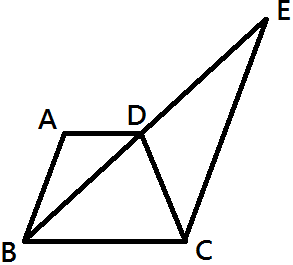# You Need To Look Everywhere

Geometry Level 3In the above figure, $ABCD$ is a trapezium, $BDE$ is a straight line and $AB \ || \ CE$ . If $BD = 8$ and $DE = 7$, find the ratio of the area of $\Delta ADB$ to the area of $\Delta CDE$.

Note: The figure is not drawn to scale.

×